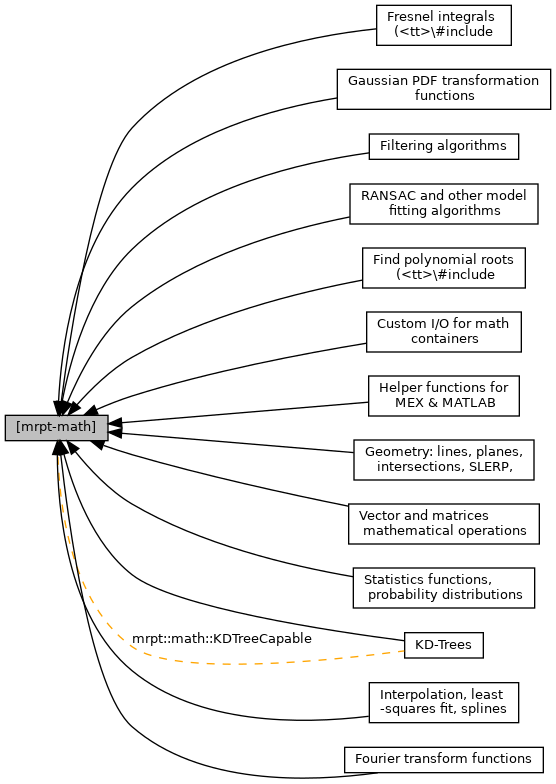MRPT  1.9.9
[mrpt-math]

## Detailed Description

Math C++ library: vectors and matrices, probability distributions, statistics, geometry, etc.

# Library mrpt-math

[New in MRPT 2.0.0]

This library is part of MRPT and can be installed in Debian-based systems with:

    sudo apt install libmrpt-math-dev


Main classes and concepts associated with this library:

Lightweight geometry entities: Write me!

Comparison: lightweight vs. {CPose*, CPoint*}: (Move to a new doc page?)

• Both can be serialized, but CPose* are CSerializable-based.
• CPose* require aligned memory (they hold Eigen containers).
• CPose* include a cache for precomputed cos/sin values, so they are preferable when doing many pose (+) point compositions.
• Lightweight containers can be constexpr constructed, CPose* cannot.
Collaboration diagram for [mrpt-math]:## Classes

class  mrpt::math::CAtan2LookUpTable
A look-up-table (LUT) of atan values for any (x,y) value in a square/rectangular grid of predefined resolution. More...

class  mrpt::math::CAtan2LookUpTableMultiRes
Like CAtan2LookUpTable but with a multiresolution grid for increasingly better accuracy in points nearer to the origin. More...

class  mrpt::math::CBinaryRelation< T, U, UIsObject >
This class models a binary relation through the elements of any given set. More...

class  mrpt::math::CHistogram
This class provides an easy way of computing histograms for unidimensional real valued variables. More...

class  mrpt::math::CLevenbergMarquardtTempl< VECTORTYPE, USERPARAM >
An implementation of the Levenberg-Marquardt algorithm for least-square minimization. More...

class  mrpt::math::CMatrix
This class is a "CSerializable" wrapper for "CMatrixFloat". More...

class  mrpt::math::CMatrixB
This class is a "CSerializable" wrapper for "CMatrixBool". More...

class  mrpt::math::CMatrixD
This class is a "CSerializable" wrapper for "CMatrixTemplateNumeric<double>". More...

class  mrpt::math::CMatrixFixedNumeric< T, NROWS, NCOLS >
A numeric matrix of compile-time fixed size. More...

class  mrpt::math::CMatrixTemplate< T >
This template class provides the basic functionality for a general 2D any-size, resizable container of numerical or non-numerical elements. More...

class  mrpt::math::CMatrixTemplateNumeric< T >
A matrix of dynamic size. More...

class  mrpt::math::CMatrixTemplateObjects< T >
This template class extends the class "CMatrixTemplate" for storing "objects" at each matrix entry. More...

class  mrpt::math::CMonteCarlo< T, NUM, OTHER >
Montecarlo simulation for experiments in 1D. More...

class  mrpt::math::CProbabilityDensityFunction< TDATA, STATE_LEN >
A generic template for probability density distributions (PDFs). More...

class  mrpt::math::CQuaternion< T >
A quaternion, which can represent a 3D rotation as pair, with a real part "r" and a 3D vector, or alternatively, q = r + ix + jy + kz. More...

class  mrpt::math::CSparseMatrix
A sparse matrix structure, wrapping T. More...

class  mrpt::math::CSparseMatrixTemplate< T >
A sparse matrix container (with cells of any type), with iterators. More...

class  mrpt::math::KDTreeCapable< Derived, num_t, metric_t >
A generic adaptor class for providing Nearest Neighbor (NN) lookup via the nanoflann library. More...

struct  mrpt::math::MatrixBlockSparseCols< Scalar, NROWS, NCOLS, INFO, HAS_REMAP, INDEX_REMAP_MAP_IMPL >
A templated column-indexed efficient storage of block-sparse Jacobian or Hessian matrices, together with other arbitrary information. More...

struct  mrpt::math::RobustKernel< KERNEL_TYPE, T >

struct  mrpt::math::RobustKernel< rkLeastSquares, T >
No robust kernel, use standard least squares: rho(r) = r^2. More...

struct  mrpt::math::RobustKernel< rkPseudoHuber, T >
Pseudo-huber robust kernel: rho(r) = 2 * delta^2 * ( -1+sqrt( 1+ r^2/delta^2 ) ) More...

## Namespaces

mrpt::math
This base provides a set of functions for maths stuff.

## Modules

Custom I/O for math containers

Statistics functions, probability distributions

Filtering algorithms

Fourier transform functions

Fresnel integrals (#include
<mrpt/math/fresnel.h>)

Geometry: lines, planes, intersections, SLERP,
"lightweight" point & pose classes

Interpolation, least-squares fit, splines

KD-Trees

Vector and matrices mathematical operations
and other utilities

Find polynomial roots (#include
<mrpt/math/poly_roots.h>)

RANSAC and other model fitting algorithms

Gaussian PDF transformation functions

Helper functions for MEX & MATLAB

## Enumerations

enum  mrpt::math::TRobustKernelType { mrpt::math::rkLeastSquares = 0, mrpt::math::rkPseudoHuber }
The different types of kernels for usage within a robustified least-squares estimator. More...

## ◆ TRobustKernelType

The different types of kernels for usage within a robustified least-squares estimator.

Use these types as arguments of the template RobustKernel<>
Enumerator
rkLeastSquares

No robust kernel, use standard least squares: rho(r)= 1/2 * r^2.

rkPseudoHuber

Pseudo-huber robust kernel.

Definition at line 23 of file robust_kernels.h.

 Page generated by Doxygen 1.8.14 for MRPT 1.9.9 Git: 7d5e6d718 Fri Aug 24 01:51:28 2018 +0200 at lun nov 2 08:35:50 CET 2020